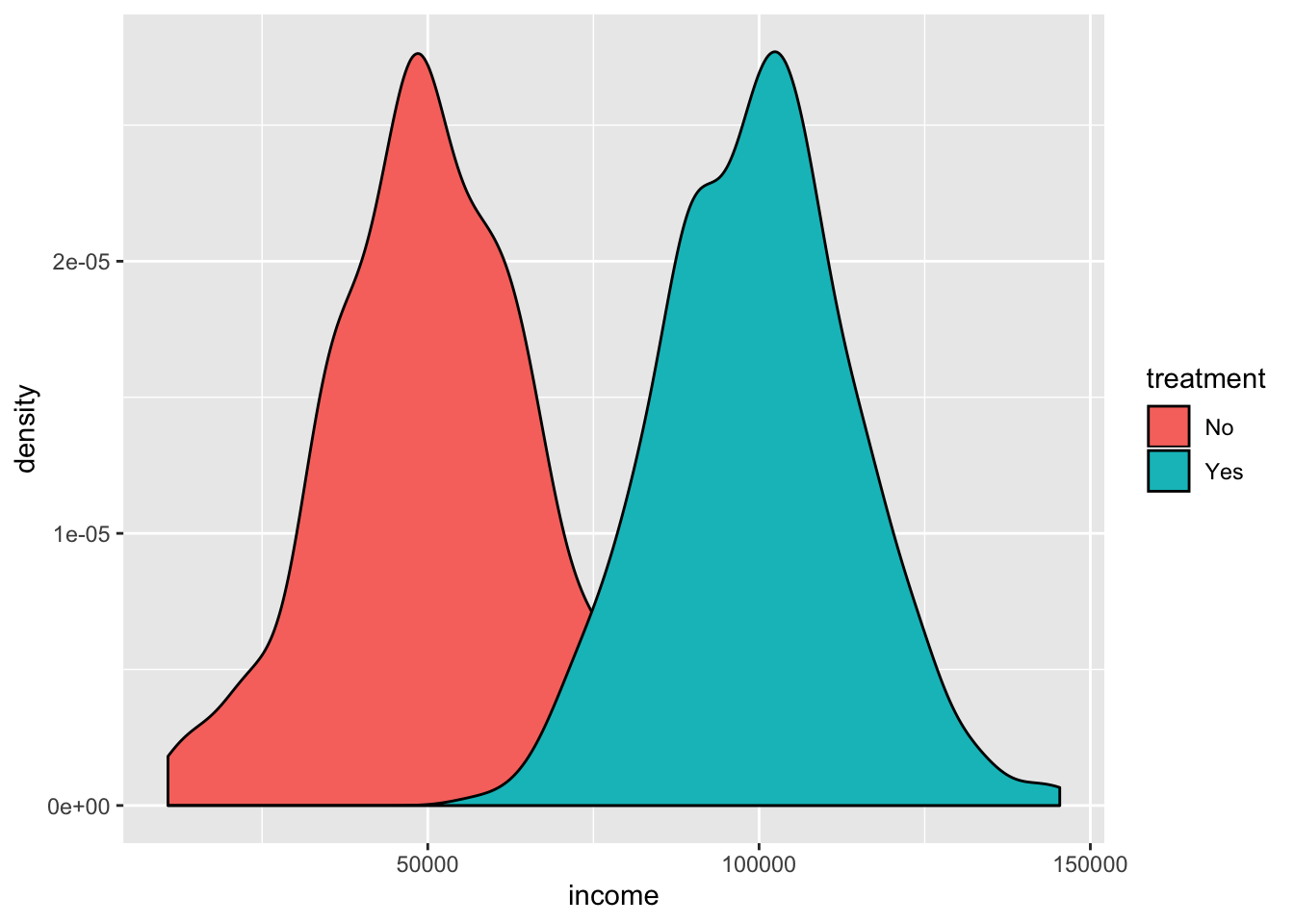# Threats to validity

Materials for class on Monday, October 7, 2019

## Generating synthetic data

In class, I briefly demonstrated how to use the wakefield package to generate synthetic data for your final project.The package is named after Andrew Wakefield, a British researcher who used fake data to create a false link between the MMR vaccine and autism.

We’ll do more work with it later in the semester, but here’s a quick example of how to use it. You can find complete documentation at GitHub.

``````library(tidyverse)
library(wakefield)

# Make all the random draws consistent
set.seed(1234)

# The r_data_frame() function lets you generate random data. You just have to
# feed it the name of a function that generates variables. You can see all the
# possible variable-generating functions by running variables() in the R
# console:
variables()``````
``````##   "age"              "animal"           "answer"
##   "area"             "car"              "children"
##   "coin"             "color"            "date_stamp"
##  "death"            "dice"             "dna"
##  "dob"              "dummy"            "education"
##  "height"           "income"           "internet_browser"
##  "iq"               "language"         "level"
##  "likert"           "lorem_ipsum"      "marital"
##  "military"         "month"            "name"
##  "normal"           "political"        "race"
##  "religion"         "sat"              "sentence"
##  "sex"              "sex_inclusive"    "smokes"
##  "speed"            "state"            "string"
##  "upper"            "valid"            "year"
##  "zip_code"``````
``````# Here we generate a small data frame. Each of the functions that are listed in variables() has arguments. See age, for instance---we can specify a range of ages to draw from
small_data <- r_data_frame(
n = 30,
age(5:18),  # Young kids
income(digits = 5),  # Rich kids
zip_code,
race,
gender_inclusive
)

# Look at the first few rows
``````## # A tibble: 6 x 5
##     Age  Income Zip   Race     Gender
##   <int>   <dbl> <chr> <fct>    <fct>
## 1    18  60519. 81105 White    Trans*
## 2     8 203525. 17611 Black    Male
## 3     8  94910. 17611 White    Female
## 4     9   4744. 95858 White    Female
## 5    12  76010. 56454 Hispanic Male
## 6     8   9534. 72651 White    Trans*``````
``````# You can make more complicated data too, like adding normally-distributed
# income, or assigning people to treatment and control groups
treatment <- r_data_frame(
n = 500,
race,
age(5:18),
income = rnorm(mean = 100000, sd = 15000)  # Normal distribution centered at 100000
) %>%
mutate(treatment = "Yes")

control <- r_data_frame(
n = 500,
race,
age(5:18),
income = rnorm(mean = 50000, sd = 15000)  # Normal distribution centered at 50000
) %>%
mutate(treatment = "No")

# We can combine these two datasets into one with bind_rows(), which essentially
# stacks the rows of one on top of the rows of the other:
big_data_set <- bind_rows(treatment, control)

# For fun, we can check the difference in income for people in the treatment and control groups
ggplot(big_data_set, aes(x = income, fill = treatment)) +
geom_density()``````Whoa! Look at that! The imaginary program boosted incomes substantially! :)

In problem set 5 you worked with fake data about a hypothetical math camp. Here’s the code I used to generate that data. The only odd thing here is the `rtruncnorm()` function, which generates data from a truncated normal distribution. This makes it so you can put limits on numbers—if you want a random distribution of GPAs centered at 3.5, you don’t want to accidentally create GPAs of 4.3 or whatever. The `a` and `b` arguments let you set a minimum and a maximum number.

``````library(tidyverse)
library(wakefield)
library(rtruncnorm)

# Make all the random draws consistent
set.seed(1234)

# Treatment group with higher post-treatment GPA
treatment <- r_data_frame(n = 794,
id,
race,
age(x = 20:30),
gender_inclusive) %>%
mutate(undergrad_gpa = round(rtruncnorm(n(), a = 1.0, b = 4.0,
mean = 2.5, sd = .5), 2),
math_camp = TRUE,
gre_verbal = round(rtruncnorm(n(), a = 130, b = 170,
mean = 145, sd = 15), 0),
gre_quant = round(rtruncnorm(n(), a = 130, b = 170,
mean = 110, sd = 15), 0),
gre_total = gre_verbal + gre_quant,
graduate_gpa = round(rtruncnorm(n(), a = 1.0, b = 4.0,
mean = 3.3, sd = .5), 2))

# Control group with slightly higher post-treatment GPA
control <- r_data_frame(n = 787,
id,
race,
age(x = 20:30),
gender_inclusive) %>%
mutate(undergrad_gpa = round(rtruncnorm(n(), a = 1.0, b = 4.0,
mean = 2.5, sd = .5), 2),
gre_verbal = round(rtruncnorm(n(), a = 130, b = 170,
mean = 145, sd = 15), 0),
gre_quant = round(rtruncnorm(n(), a = 130, b = 170,
mean = 110, sd = 15), 0),
math_camp = FALSE,
gre_total = gre_verbal + gre_quant,
graduate_gpa = round(rtruncnorm(n(), a = 1.0, b = 4.0,
mean = 2.9, sd = .5), 2))

population <- r_data_frame(n = 1986,
id,
race,
age(x = 20:30),
gender_inclusive) %>%
mutate(undergrad_gpa = round(rtruncnorm(n(), a = 1.0, b = 4.0,
mean = 2.5, sd = 1.5), 2),
gre_verbal = round(rtruncnorm(n(), a = 130, b = 170,
mean = 145, sd = 15), 0),
gre_quant = round(rtruncnorm(n(), a = 130, b = 170,
mean = 160, sd = 30), 0),
math_camp = FALSE,
gre_total = gre_verbal + gre_quant,
graduate_gpa = round(rtruncnorm(n(), a = 1.0, b = 4.0,
mean = 3.6, sd = 1), 2))

# Combine them all
experiment <- bind_rows(treatment, control) %>%
sample_frac(1)  # Shuffle the dataset for kicks and giggles

everyone <- bind_rows(experiment, population) %>%
sample_frac(1)

# Save these as CSV files
write_csv(experiment, "math_camp_experiment.csv")
write_csv(everyone, "math_camp_everyone.csv")``````

## Clearest and muddiest things

Go to this form and answer these three questions:

1. What was the muddiest thing from class today? What are you still wondering about?
2. What was the clearest thing from class today?
3. What was the most exciting thing you learned?

I’ll compile the questions and send out answers after class.